# Can we short JFET source and gate junctions as shown in the circuit?

#### skyline977

Joined Jan 14, 2021
29
Hi,
Is the below circuit accurate?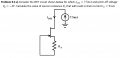Because we know that id = is, however here there seems no path for that. Also, ig = 0 always which contradicts with the way the circuit is done.
In my humble opinion I suspect it's as I draw it below: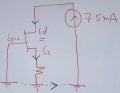Am I right or not?

#### crutschow

Joined Mar 14, 2008
31,544
Am I right or not?
Don't completely understand what you said but I think, mostly not.

The gate-source voltage (Vgs) will be reverse biased by the voltage drop across Rs due to the current through it from the transistor drain-source current.
A JFET conducts it's maximum drain-source current (IDSS) when when Vgs = 0V and a negative Vgs will start to turn it off.
So from that you can solve the problem.

#### neonstrobe

Joined May 15, 2009
182
I think you are on the right tracks, actually. Yes, the drain current is the same as the source current and there is no gate current except for a very low level of leakage.
The arrow on the gate indicates a diode and that the gate is the anode or p-type. So it is not conducting, as you say, because it is either below the forward bias voltage (0.6V) or reversed because the source voltage is higher.

•skyline977

#### skyline977

Joined Jan 14, 2021
29
Don't completely understand what you said but I think, mostly not.

The gate-source voltage (Vgs) will be reverse biased by the voltage drop across Rs due to the current through it from the transistor drain-source current.
A JFET conducts it's maximum drain-source current (IDSS) when when Vgs = 0V and a negative Vgs will start to turn it off.
So from that you can solve the problem.
The way the circuit is drawn in the question looks like the source current is entering the gate, which is not possible.
Also, how the source current would return to the drain in the way it's drawn in the question there is no ground nor a return path from the source back to the drain right?

#### skyline977

Joined Jan 14, 2021
29
I think you are on the right tracks, actually. Yes, the drain current is the same as the source current and there is no gate current except for a very low level of leakage.
The arrow on the gate indicates a diode and that the gate is the anode or p-type. So it is not conducting, as you say, because it is either below the forward bias voltage (0.6V) or reversed because the source voltage is higher.
Also, the circuit drawn is confusing, because there should be a return path from the source current back to the drain to be a close or complete circuit.

#### crutschow

Joined Mar 14, 2008
31,544
Also, the circuit drawn is confusing, because there should be a return path from the source current back to the drain to be a close or complete circuit.
Yes, the bottom of Rs should be connected to circuit common (ground).

Also the drain supply should be a voltage, not a current source.
With an ideal current source the current will always be 7.5mA regardless of the value of Rx or anything else.

•skyline977

#### skyline977

Joined Jan 14, 2021
29
Yes, the bottom of Rs should be connected to circuit common (ground).

Also the drain supply should be a voltage, not a current source.
With an ideal current source the current will always be 7.5mA regardless of the value of Rx or anything else.

Ok. THANKS

Also the drain supply should be a voltage, not a current source.
With an ideal current source the current will always be 7.5mA regardless of the value of Rx or anything else.

#### MrAl

Joined Jun 17, 2014
9,781
Hello,

Am i missing something here?
Except for the implied (missing) ground and the current source instead of a voltage source of the original circuit it looks like it should work.

The reason for this is because the resistor provides source feedback, which is negative feedback, so the circuit should reach a state of equilibrium and therefore stay fixed at one current level.
The current does not have to flow though the gate i am not sure why anybody would think that it would.

As to the feedback mechanism, consider what happens at the source as current increases from say 100ua on up (to maybe 10ma or something like that with a voltage source as the power source not a current source). The source becomes more and more positive simply because the source resistor drops more and more voltage as the current through that resistor increases. Since the gate is grounded, it is at 0 volts, so the gate source junction becomes more and more reverse biased. As it becomes more and more reverse biased it passes less and less current. The limiting point is the equilibrium point where the reverse voltage becomes equal to the gate source voltage needed to pass the level of the current through the resistor that produces that reverse voltage.
A quick example would be with a 100 Ohm resistor with 1000uf in parallel where the source current is 10ma producing 1 volt of reverse bias, and that 1 volt just happens to be the gate to source voltage (which would then be considered -1 volt) that produces 10ma through the drain.
Now consider what happens before we reach that 1v. At 0.5v, the current would be higher (some going through the capacitor) but as the capacitor charges up the resistor passes all the current so the gate to source voltage starts to go higher (toward that 1v). Once it reaches 1v (-1v) the current is 10ma and 10ma through 100 Ohms produces 1v so that is the end of the transition period and the circuit becomes stable. Thus, by choosing the source resistor value we can set the current (to some limit determined by the minimum resistance of the drain to source). .

So really this is very similar to an NPN with an emitter feedback resistor. Should not be much of a mystery. Of course seeing a current source where normally we would have a voltage source, that's a big mystery.

•skyline977

#### MrAl

Joined Jun 17, 2014
9,781
Here are two circuits where we might actually be able to use a current source. We need some way to divert some of the current away from the FET so a resistor would be a possibility. We only have to know the gate voltage required to cause 5ma of drain current to solve these, if solutions do in fact exist.
This makes it a little more interesting#### Attachments

•skyline977

#### skyline977

Joined Jan 14, 2021
29
Here are two circuits where we might actually be able to use a current source. We need some way to divert some of the current away from the FET so a resistor would be a possibility. We only have to know the gate voltage required to cause 5ma of drain current to solve these, if solutions do in fact exist.
This makes it a little more interestingThank you you made it very clear. I really appreciate your explanation. Thanks again,

#### MrAl

Joined Jun 17, 2014
9,781
Thank you you made it very clear. I really appreciate your explanation. Thanks again,
You are welcome. Maybe we can try those circuits next just for the exercise.
We know right off that the added Rds or added Rd has to pass 2.5ma so that helps right away.

#### Jony130

Joined Feb 17, 2009
5,439
First, you need to find the Vgs voltage that will ensure 5mA at the drain.

$I_D =I_{DSS}\left(1 - \frac{V_{GS}}{V_T}\right)^2$

As you have noticed we have a two solution:

Vgs = -5.449V and Vgs = -0.55V but only one has a physical meaning. Do you know which one? And why this one?

•skyline977

#### skyline977

Joined Jan 14, 2021
29
First, you need to find the Vgs voltage that will ensure 5mA at the drain.

$I_D =I_{DSS}\left(1 - \frac{V_{GS}}{V_T}\right)^2$

As you have noticed we have a two solution:

Vgs = -5.449V and Vgs = -0.55V but only one has a physical meaning. Do you know which one? And why this one?
I GOT IT. Thank you.
Yes, Vgs = -0.55V, because Vgs must be greater than Vt so as not to be in cutoff region.
By KVL we have:
+Vgs + RS*ID = 0,
RS = -Vgs/ID
Rs = -(-0.55V) / 5mA = + 110 Ω
Now we have to find Vgd
+Vds + Is*Rs = 0,
Vds = - 0.55V

Vgd = Vgs- Vds = -0.55 -(-0.55)
Vgd = 0 V
Vgd < Vt
Therefore, our assumption was wrong. The JFET is in triode mode below is the recalculation: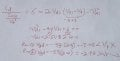I think the above approach is wrong, I think the below approach is the correct one, however, I am stuck AGAIN with a negative resistor, where have I gone wrong?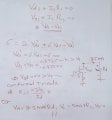Thank you.

Last edited:

#### skyline977

Joined Jan 14, 2021
29
First, you need to find the Vgs voltage that will ensure 5mA at the drain.

$I_D =I_{DSS}\left(1 - \frac{V_{GS}}{V_T}\right)^2$

As you have noticed we have a two solution:

Vgs = -5.449V and Vgs = -0.55V but only one has a physical meaning. Do you know which one? And why this one?
OK, below is my final attempt I KNOW it's wrong but not sure where is my mistake! Please help.

Thanks again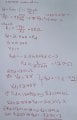#### skyline977

Joined Jan 14, 2021
29
You are welcome. Maybe we can try those circuits next just for the exercise.
We know right off that the added Rds or added Rd has to pass 2.5ma so that helps right away.
Hi,
Can you kindly review my 3 different attempts I KNOW There is a mistake, but WHERE

#### MrAl

Joined Jun 17, 2014
9,781
Hi,
Can you kindly review my 3 different attempts I KNOW There is a mistake, but WHERE
Ok i will.

In the mean time, i was looking at the circuit on the right in post #9.
If we remove Rd and replace the 7.5ma current source with a 5ma current source we have almost the same problem circuit just minus the as of yet uncalculated resistor voltage for Rd.

With that, we note that we can make the value of Rs anything. That means that the reverse gate voltage can be just about anything. But even if we choose a particular Rs value like 100 Ohms, it may be hard to calculate Vd the voltage measured drain to ground. We'd have to use a more advanced model equation that includes Vds. But even then, can we really calculate Vds? I think we may need an even more advanced model to calculate that one that approaches a full spice model.

#### MrAl

Joined Jun 17, 2014
9,781
Hi,
Can you kindly review my 3 different attempts I KNOW There is a mistake, but WHERE
Hello again,

What circuit(s) are you working with? Please show a drawing here rather than a referral to a previous drawing in this thread or some other location.
Also, what do you use for Vp (or as it is sometimes called VT or VTO)?
Also, what do you use for Idss at Vgs=0 ?

Vp and Idss have to be known or solved for given some measurements.
For example, using the U209 NJFET included with LTSpice i get values of:
Vp=-2 volts
Idss=-0.023 amps

and i get reasonable results that somewhat match a simulation.
This of course leads to different results than anyone else got here and that is simply because it is a different JFET device and they are all a little different so a different JFET model will lead to different results of course because the intrinsic parameters are different.
Sine there is also a variation in Id with Vds and i wanted to avoid that here, i used the average value of Id for Vds=1 and Vds=10 volts in order to calculate Vp. This worked out reasonably well for a model that does not include Vds. As it turned out, the value i got for Vp was very close to the default spice value for Vp which is -2 volts so i used -2 volts for Vp.
Note also that all the values i used were negative. That includes Vp, Vgs, Id, and Idss also, but Vds was kept positive. This allowed me to use the simpler model for the JFET:
Id=Idss*(1-Vgs/Vp)^2
where
Idss is extracted for Vgs=0 volts and is also negative.
It is most likely possible to turn all of these positive, because Vgs/Vp will always be positive if both are negative, and if Idss is positive then Id will be positive. I kept them negative because LTSpice produced negative values (due to the way i measured Id).
Also note these values were found using the raw device itself with no resistors in the circuit which meant setting Vgs with a separate (negative) voltage source and a positive voltage source for Vds which i allowed to vary from 1 to 10 volts for parameter extraction and testing.
Note that in real life Vgs and Vp will be negative and Idss and Id will be positive.

Last edited: